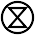### Find Logarithm and Anti-logarithm

Script to find natural logarithm and anti-logarithm of given number.

The logarithm of a number is the exponent by which another fixed value, the base, has to be raised to produce that number. For example, the logarithm of 1000 to base 10 is 3, because 1000 is 10 to the power 3: 1000 = 103 = 10 × 10 × 10. More generally, if x = by, then y is the logarithm of x to base b, and is written logb(x), so log10(1000) = 3.
- Wikipedia ( http://en.wikipedia.org/wiki/Logarithm )

The natural logarithm is the logarithm to the base e, where e is an irrational and transcendental  constant approximately equal to 2.718281828. The natural logarithm is generally written as ln(x), loge(x) or sometimes, if the base of e is implicit, as simply log(x)
-Wikipedia ( http://en.wikipedia.org/wiki/Natural_logarithm )

-------------------------------------------------------------------------------------------------------------------------------------------------
HOW THIS SCRIPT WORKS:
bc is an awesome command line calculator.In bc one -liner for finding log of 3 is echo "l(3)" | bc -l .
The script makes it easier to do the same with very little typing.
-------------------------------------------------------------------------------------------------------------------------------------------------
-------------------------------------------------------------------------------------------

#!/bin/bash
# to find the natural logarithm of given number

#for finding logarithm continuously
if [[ \$1 == \$null ]];then
do
if [[ \$num != \$null ]];then
log=\$(echo "scale=3; l(\$num)" | bc -l)
echo -e "\e[1;34m\$log\e[0m"
fi
done
exit 0
fi

#for finding logarithm of a single number
log=\$(echo "scale=3; l(\$1)" | bc -l)
echo -e "\e[1;34m\$log\e[0m"

-------------------------------------------------------------------------------------------

In computer the script is named log . To find logarithm of say 2 , in terminal just type log 2 and press enter ..

But suppose you want logarithm of several number, then it is irritating to type log all the time. For that purpose  type  log ones and press enter .After that type any number of numbers pressing enter after each one to get logrithm  ..

Press ctrl D or ctrl C to exit.

Now we move to script on anti-logarithm ..

Anti-logrithm is the number for which a given logarithm stands; for example, where log x equals y, then x is the antilogarithm of y

-------------------------------------------------------------------------------------------------------------------------------------------------
HOW THIS SCRIPT WORKS:
In bc one -liner for finding anti log of 3 is echo "e( 3*l(2.71281828))" | bc -l
The script makes it easier to do the same with very little typing.
-------------------------------------------------------------------------------------------------------------------------------------------------
-------------------------------------------------------------------------------------------
#!/bin/bash
#script for finding the anti-logarithm

#for finding anti-logarithm continuously
if [[ \$1 == \$null ]];then
do
if [[ \$num != \$null ]];then
antilog=\$(echo "scale=3; e(\$num*l(2.7182818284590))" | bc -l)
echo -e "\e[1;34m\$antilog\e[0m"
fi
done
exit 0
fi

#for finding anti-logarithm of a single number
antilog=\$(echo "scale=3; e(\$1*l(2.7182818284590))" | bc -l)
echo -e "\e[1;34m\$antilog\e[0m"

-------------------------------------------------------------------------------------------

In my computer the script is named antilog . Works similar as the log script ..

and..

YAHOO .....

Enjoy Linux !!

Don't know what to do with codes ?? click here

#### 1 comment:

1....why does your antilog script multiply your \$num by the natural log of e, i.e., 1? Wouldn't e(\$num) be far more concise and clear to readers, not to mention more-consistent with the simple l(\$num) syntax you used above?